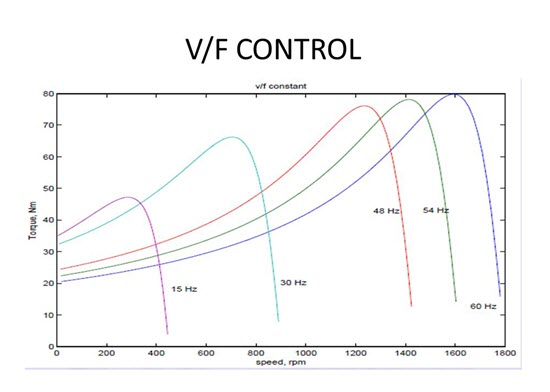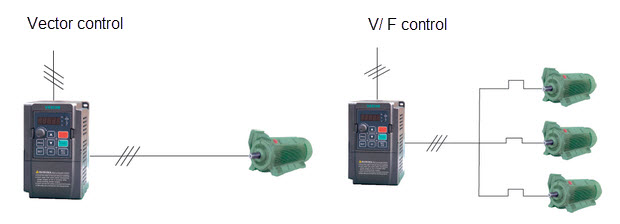An introduction about V/F control like its control principle, applications, the pros and cons of V/F control of induction motor.

When it refers to electric motor control modes, V/F control algorithm is one of the basic control modes which is widely used in pump and fan system. In this post, we will introduce the control principle, application and more about VF control method.

### 1. Introduction about V/F Control

V/F is abbreviated from voltage/frequency. V/F control is an induction motor control method which ensures the output voltage proportional with the frequency, so it maintains a constant motor flux, preventing weak magnetic and magnetic saturation phenomenon from happening.

### 2. Control Principle

V/F control principle is to produce a circuit called voltage-controller oscillator with oscillator frequency. It is a voltage-dependent capacitance, when subjected to a change in voltage, its capacity will change, and then the change in capacity will cause changes in the oscillation frequency, resulting in variable frequency. This controlled frequency is used to control the frequency of the output voltage, in order to achieve speed changes of the controlled electric motors.### 3. Applications of V/F Control

Asynchronous electric motor torque is a result of the interaction of flux and rotor flux. At a rated frequency, if voltage is set to a certain value and only reduce the frequency, then there will be large magnetic flux and magnetic circuit saturation (severely, it will burn motor). Therefore, the frequency and voltage must be changed proportionally. When changing the frequency, we should control the output voltage of AC drive, in order to keep constant flux and avoid weak magnetic and magnetic saturation phenomenon. This control method is commonly applied for fans and pumps.

### 4. Pros and Cons of V/F Control

Currently, most of the general purpose are using the V/F control method. The VFDs which are of V/F control usually features simple structure. But this kind of VFD adopts open-loop control, so it is difficult to achieve high control performance, moreover, at low frequency, it requires for torque compensation in order to change the low frequency torque characteristics.

The below image shows the motor control modes supported by Veichi AC70: V/F control and vector motor control mode: